# How to get Text & Number in Reverse through VBA in Microsoft Excel

In excel, there are times when we want to get the text reversed completely or their order with VBA code. There can be various requirements, like extracting reverse cell content, reverse cell order & so on.

• How to get reverse cell content?
• How to get all words in reverse order from a cell?
• How to reverse column order?
• How to get reverse numbers only from text?
• How to reverse cell content of activecell?

How to get reverse cell content?

In Excel there are requirement to reverse the text or numbers in cells e.g. “english” to  “hsilgne”

Following is the snapshot of data before output: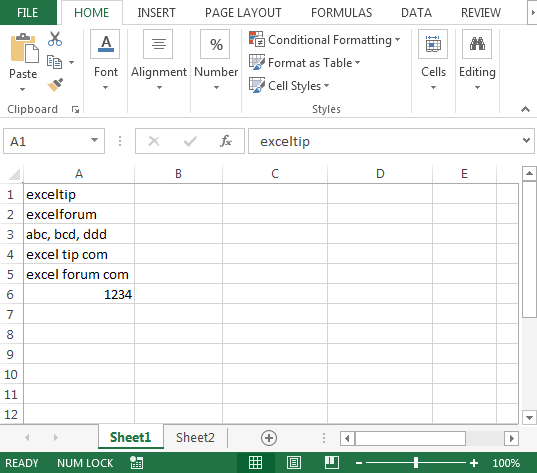Following is the snapshot of require output in column B: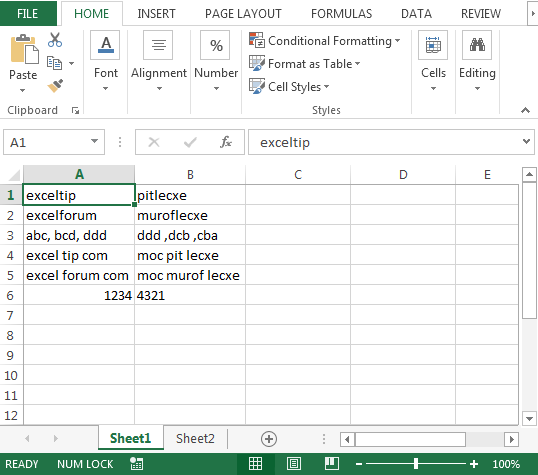To get the above output, we need to follow the below steps to launch VB editor

• Click on Developer tab
• From Code group, select Visual Basic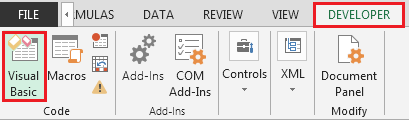• Copy the below code in the standard module

```Function CompleteReverse(rCell As Range, Optional IsText As Boolean)
Dim i As Integer
Dim StrNewTxt As String
Dim strOld As String
strOld = Trim(rCell)
For i = 1 To Len(strOld)
StrNewTxt = Mid(strOld, i, 1) & StrNewTxt
Next i
If IsText = False Then
CompleteReverse = CLng(StrNewTxt)
Else
CompleteReverse = StrNewTxt
End If
End Function
```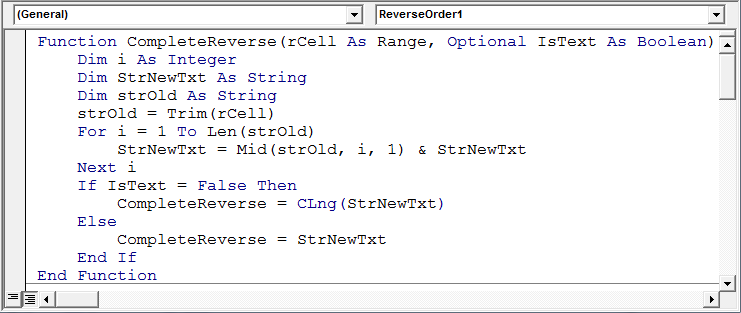• In cell B1 the formula will be
• =CompleteReverse(A1,TRUE)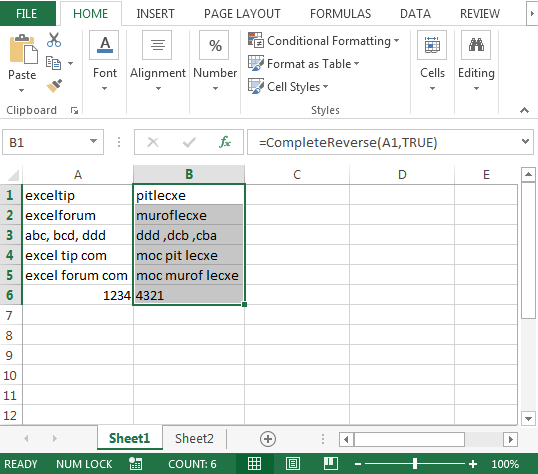How to get all the words in reverse order from a cell?

We will have couple of codes to find the solution. To get all words in complete reverse order, we will copy & paste the following code in module

```Function ReverseOrder1(Rng As Range)
Dim Val As Variant, Counter As Integer, R() As Variant
Val = Split(Application.WorksheetFunction.Substitute(Rng.Value, " ", ""), ",")
ReDim R(LBound(Val) To UBound(Val))
For Counter = LBound(Val) To UBound(Val)
R(UBound(Val) - Counter) = Val(Counter)
Next Counter
ReverseOrder1 = Join(R, ", ")
End Function
```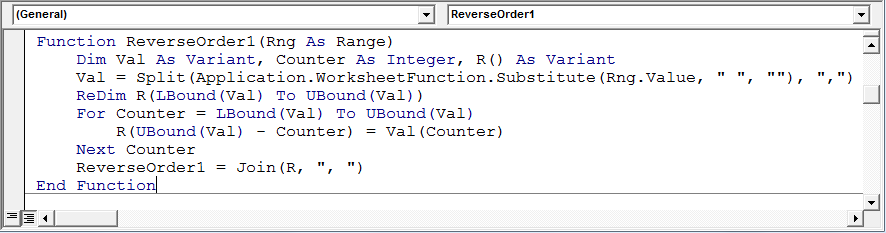• In cell C1 the formula will be
• =ReverseOrder1(A1)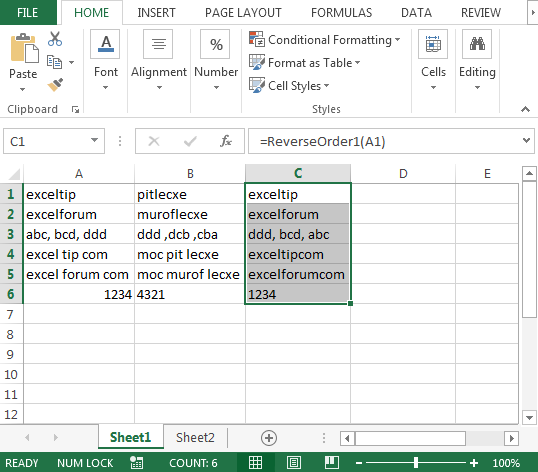Let’s take a look at second VBA code:

```Function ReverseOrder2(Rng As Range) As String
Dim Counter As Long, R() As String, temp As String
R = Split(Replace(Rng.Value2, " ", ""), ",")
For Counter = LBound(R) To (UBound(R) - 1) \ 2
temp = R(UBound(R) - Counter)
R(UBound(R) - Counter) = R(Counter)
R(Counter) = temp
Next Counter
ReverseOrder2 = Join(R, ", ")
End Function
```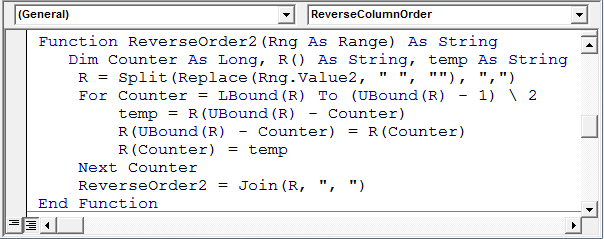• In cell D1 the formula will be
• =ReverseOrder2(A1)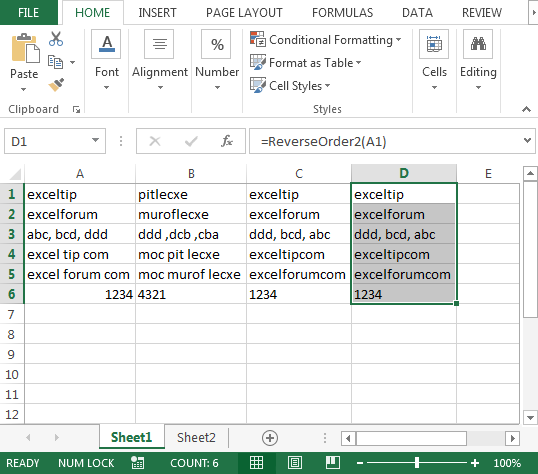How to reverse column order?

In case you have a requirement to reverse the order of a column data then you should have a closer look at the below code:

```Sub ReverseColumnOrder()
Dim wBase As Worksheet, wResult As Worksheet, i As Long, x As Long
Set wBase = Sheets("Sheet1")
Set wResult = Sheets("Sheet2")
Application.ScreenUpdating = False
With wBase
For i = .Range("A1").CurrentRegion.Rows.Count To 1 Step -1
x = x + 1
.Range("A1").CurrentRegion.Rows(i).Copy wResult.Range("A" & x)
Next i
End With
Application.ScreenUpdating = True
End Sub
```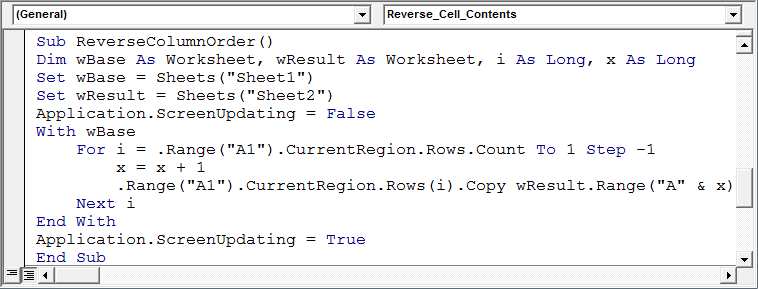The above code will check the data in column A in sheet1 & then reverse the order in sheet 2. Refer below image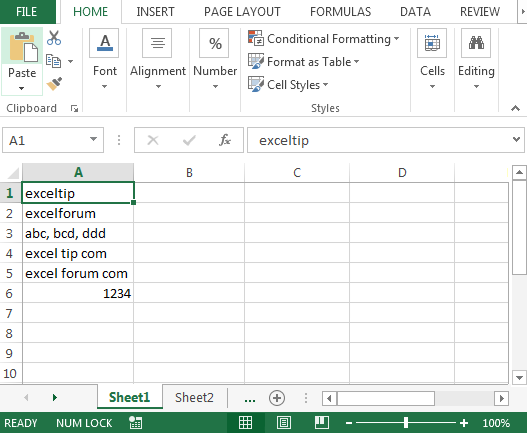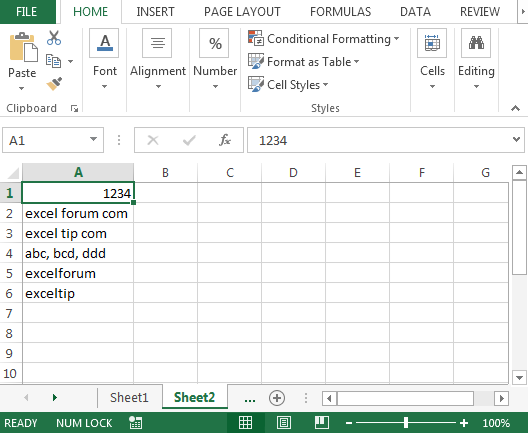How to get reverse numbers only from text?

Example: “excel (123) tip” is the cell content

Require output: “excel (321) tip”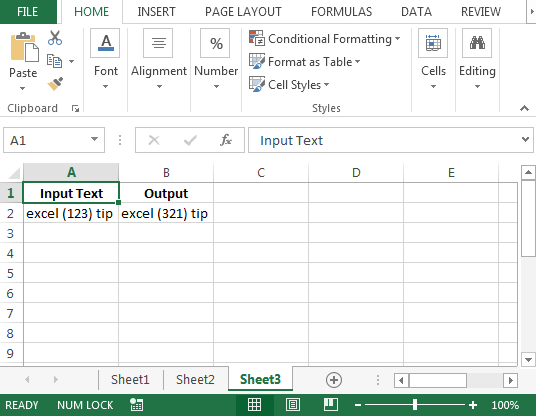In excel, there can be multiple ways to get the same output & same goes to finding solution with VBA UDF’s. For this example, we will show 5 different ways.

Copy & paste the following codes in standard module:

```Function ReverseNumber1(v As Variant) As String
Dim iSt As Integer, iEnd As Integer, sNum As String, sTemp As String
iSt = InStr(v, "(")
iEnd = InStr(v, ")")
If iSt = 0 Or iEnd = 0 Then ReverseNumber1 = v: Exit Function
sNum = Mid(v, iSt + 1, iEnd - iSt - 1)

For i = Len(sNum) To 1 Step -1
sTemp = sTemp & Mid(sNum, i, 1)
Next i
ReverseNumber1 = Left(v, iSt) & sTemp & Mid(v, iEnd, 5 ^ 5)
End Function

Function ReverseNumber2(s As String) As String
Dim i&, t\$, ln&
t = s: ln = InStr(s, ")") - 1
For i = InStr(s, "(") + 1 To InStr(s, ")") - 1
Mid(t, i, 1) = Mid(s, ln, 1)
ln = ln - 1
Next
ReverseNumber2 = t
End Function

Function ReverseNumber3(c00)
c01 = Split(Split(c00, ")")(0), "(")(1)
ReverseNumber3 = Replace(c00, "(" & c01 & ")", "(" & StrReverse(c01) & ")")
End Function

Function ReverseNumber4(c00)
ReverseNumber4 = Left(c00, InStr(c00, "(")) & StrReverse(Mid(Left(c00, _
InStr(c00, ")") - 1), InStr(c00, "(") + 1)) & Mid(c00, InStr(c00, ")"))
End Function

Function ReverseNumber5(s As String)
Dim m As Object
With CreateObject("VBScript.Regexp")
.Global = True
.Pattern = "(\D*)(\d*)"
For Each m In .Execute(s)
ReverseNumber5 = ReverseNumber5 & m.submatches(0) & StrReverse(m.submatches(1))
Next
End With
Set m = Nothing
End Function
```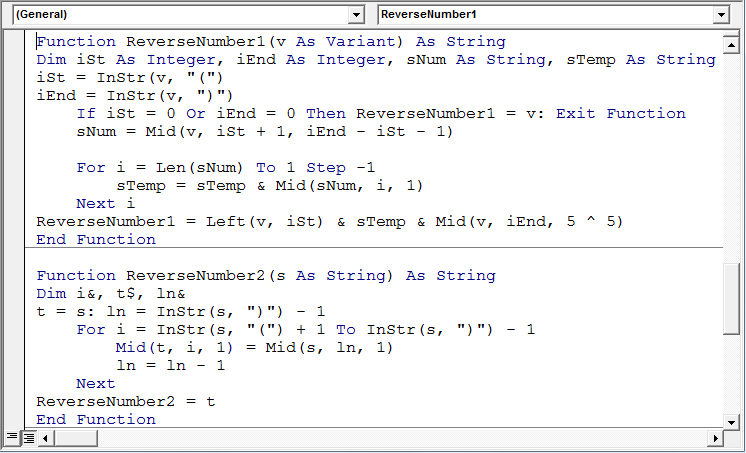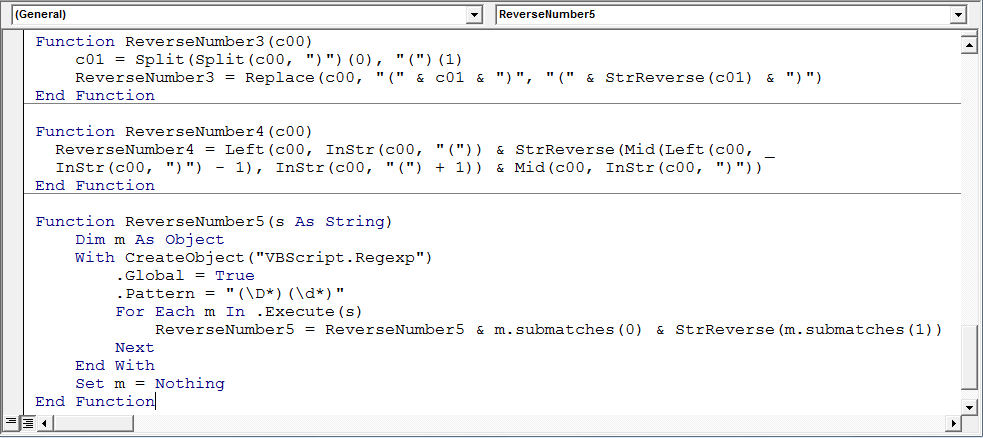• In cell B2, the formula will be
• =ReverseNumber1(A2)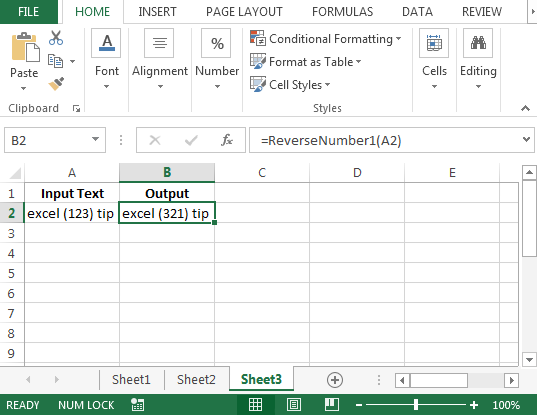We can test the other 4 codes with the following formula:

1. =ReverseNumber2(A2)

2. =ReverseNumber3(A2)

3. =ReverseNumber4(A2)

4. =ReverseNumber5(A2)

All of the above 5 macro codes will provide the same output; however; one can adopt the code they are most comfortable with.

How to reverse cell content of activecell?

In case you want a macro to run on activecell only & then reverse the content. This code will not run on cell which contains formula.

We will use the following code:

```Sub Reverse_Cell_Contents()
'this macro will run only on activecell' --- Comment
If Not ActiveCell.HasFormula Then
sRaw = ActiveCell.Text
sNew = ""
For j = 1 To Len(sRaw)
sNew = Mid(sRaw, j, 1) + sNew
Next j
ActiveCell.Value = sNew
End If
End Sub
```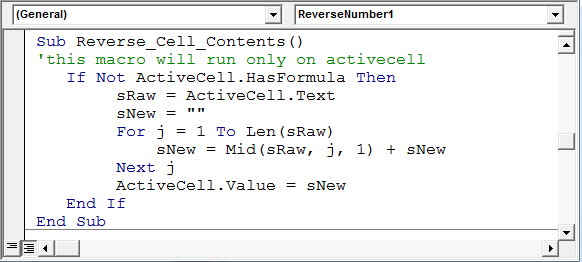If the cursor is on cell A1, which contains “exceltip”, then the above macro will convert it into “pitlecxe”.

Conclusion: We can have as many UDFs for a single solution in Microsoft Excel. This UDF will work from 2003 version to 2013.